American Journal of Theoretical and Applied Statistics
Volume 4, Issue 4, July 2015, Pages: 264-268

Study on Financial Market Risk Measurement Based on Asymmetric Laplace Distribution

Hong Zhang, Li Zhou, Jie Zhu

School of Information, Beijing Wuzi University, Beijing, China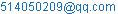(Hong Zhang)

Hong Zhang, Li Zhou, Jie Zhu. Study on Financial Market Risk Measurement Based on Asymmetric Laplace Distribution. American Journal of Theoretical and Applied Statistics. Vol. 4, No. 4, 2015, pp. 264-268. doi: 10.11648/j.ajtas.20150404.16

Abstract: In this paper, According to the returns distributions (of the financial assets returns series) with peak fat-tailed and asymmetric and the theory of Asymmetric Laplace distribution. AL-VaR (AL-CVaR) parametric method and Monte Carlo simulation are proposed which are based on Asymmetric Laplace distribution. We analyze the VaR (CVaR) measuring model of AL distribution and discuss its backtesting. And then we evaluate the pros and cons of each method combining with the characteristics of the stock market risk of three countries. (America, China and Japan).

1. Introduction

In the paper, we analyze the VaR (CVaR) measuring model of AL distribution and discuss its backtesting. And then we evaluate the pros and cons of each method combining with the characteristics of the stock market risk of three countries. (China Japan and America).

In order to better capture the market risk features such as biased and thick tail, to study the distribution of the risk further. Balakrishnan and Basu (1995)1, Bain and Engelhardt (1973)2, Kotz et al. (2001, 2002)3-5,Trindade and Zhu (2007)6-7 have done a lot of research on non symmetric Laplasse (Asymmetric Laplace, AL) family of distributions.

Trindade and Zhu (2007) studied progressive distribution of financial risk estimates based on the AL distribution. Jayakumar and Kuttykrishnan (2007)8 and Trindade et al. (2010) studied the model of time sequence based on AL distribution.AL distribution can fit the data characteristics well of Asymmetric and thick tail.

There are 3 Levels titles in an article to make ideas clear:

(1) Given fitting test of AL distribution and empirical analysis of risk measurement.

(2) Given the market risk value (VaR and CVaR ) and its backtesting.

(3) Proposing ErrorVaR and ErrorCVaR for analyzing the effectiveness of methods9-11.

2. The Empirical Analysis

2.1. The Selection of Data and Its Characteristics

Selecting S.H.I (Shanghai composite index), Nikkie225 and S&P500 as research objects. Sample interval is from 2010.01.04 to 2014.12.31. Using Logarithm yields,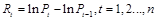The results of statistics (table 1) show that tail of exponential gains and losses distribution is fatter than normal distribution’s. Which mains abnormal fluctuations in the market happen sometimes, the fact that skewness is all negative shows, from a long-term perspective, that fluctuation in the left side of exponential gains and losses distribution is larger than right side. So normal distribution cannot effectively characterize these phenomena. Stationary ADF-test results that H=1and P=1.0e-0.3 are far less than 0.05, showing the results reject unit root process hypothesis, and accept the hypothesis of stationary sequence. Simultaneously, indexes are tested by normal J-B .Results that H=1 reject normal distribution hypothesis. Known from the analysis, financial time series usually have some obvious characteristics. The normal distribution assumption commonly is not truly reflecting the real situation of the fluctuation of reality. The test of AL distributions are listed. (Table.1)

2.2. Al Distribution Fitting Text

Maximum likelihood estimates of the parameters of AL distribution (each market index) can be calculated. (Table.2) Thus, we assume that each return series obey the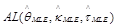distribution corresponding. Fitting histograms are listed respectively with the AL density function of S.H.I, Nikkie225 and S&P500. Known from tables, characteristics of financial data samples can be well fitted by AL distribution, such as excess kurtosis, fat tail and asymmetry. K-S test results of AL distribution (of returns in each market index) show: There are all H=0, and P>0.05.So in 5% or 1% significance level, and various market indices have accepted hypothesis that the sample data is subject to AL distribution13,15.

Table 1. Yield-related statistics and its stationarity test results.

 Yield Mean Variance Skewness Kurtosis ADF-test S.H.I 0.0079 0.0004 -0.3469 5.4187 1(1.0e-3) Nikkie225 -0.0001 0.0002 -0.4456 11.2579 1(1.0e-3) S&P500 -0.0001 0.0001 -0.2481 13.2471 1(1.0e-3)

Notes: H value and P value in ADF-test

Table 2. Each distribution parameter estimation and distribution test.

 Yield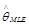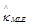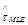K-S text S.H.I 0.0040 1.1118 0.0255 0(0.8521) Nikkie225 0.0013 1.0555 0.0064 0(0.8254) S&P500 0.0014 1.0000 0.0131 0(0.1359)

Notes: H value and P value in K-S test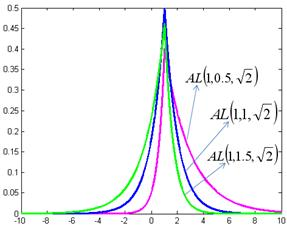Figure 1. AL density function fitting.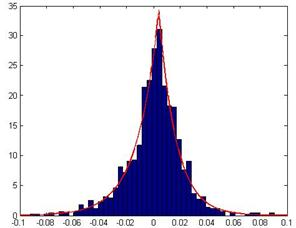Figure 2. AL density function fitting of S.H.I.

(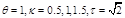)Figure 3. AL density functions fitting of Nikkie225.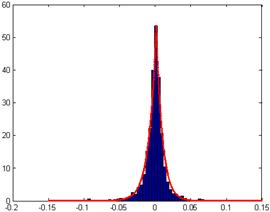Figure 4. AL density function fitting of S&P500.

2.3. Calculating VaR and CVaR

2.3.1. Al-Var Parametric Method and Al-Cvar Parametric Method Theorem

Suppose Y is the profits and losses of a certain financial portfolio, Y is a random variable ,obeying Asymmetric Laplace distribution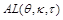,for a given confidence level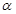(Usually ranging from 95% to 99% ):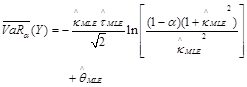(1)

The theoretical value of VaR and CVaR respectively is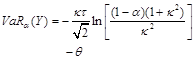(2)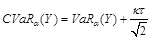(3)

(2)Maximum likelihood estimate of VaR and CVaR respectively is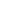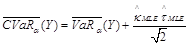(4)

The estimated values of market risk, VaR and CVaR calculated by using theorem are listed.(Table 3)

2.3.2. Al-Mc Method

Using the AL-MC method generate random number which are subject todistribution correspondingly to calculate the values of   the market risk, VaR and CVaR. (See Table 3)

Table 3. The results of backtesting and accuracy evaluation of VaR and CvaR.

 Confidence level 95% 97.5% N-VaR S.H.I 0.0330(1.1459) 0.0340(8.7931) Nikkie225 0.0290(2.4001) 0.0355(0.1646) S&P500 0.0249(1.3902) 0.0258(2.1854) ErrorVaR 0.6214E-004 0.8412E-004 N-CVaR S.H.I 0.0422[0.0070] 0.0465[0.0054] Nikkie225 0.0354[0.0148] 0.0409[0.0145] S&P500 0.0356[0.0108] 0.0356[0.0111] ErrorCVaR 0.9546E-004 1.3035E-004 Parameter Value Par AL-VaR S.H.I 0.0412(9.2978) 0.0515(7.1552) Nikkie225 0.0299(3.5000) 0.0354(0.0547) S&P500 0.0250(1.3956) 0.0345(0.0082) ErrorVaR 1.7456E-004 0.4152E-004 AL-CVaR S.H.I 0.0601[-0.0025] 0.0714[-0.0025] Nikkie225 0.0411[0.0069] 0.0521[0.0014] S&P500 0.0348[00.0071] 0.0147[0.0090] ErrorCVaR 0.3456E-004 0.4841E-004 Non-Parameter Value AL-VaR S.H.I 0.0325(0.0752) 0.0465(0.0025) Nikkie225 0.02483(0.5419) 0.0352(0.0525) S&P500 0.0248(0.6328) 0.0258(3.9541) ErrorVaR 0.1549E-004 0.2858E-004 AL-CVaR S.H.I 0.0522[-0.0020] 0.0625[-0.0035] Nikkie225 0.0341[0.0059] 0.0145[0.0025] S&P500 0.0326[0.0058] 0.0325[0.0065] ErrorCVaR 0.2400E-004 0.4078E-004

Table 3. continue.

 Confidence level 99% 99.5% 99.9% N-VaR S.H.I 0.0423(15.3001) 0.0545(22.2541) 0.0654(24.5945) Nikkie225 0.0452(8.8152) 0.0452(19.6541) 0.0587(33.4852) S&P500 0.0358(8.3012) 0.0395(23.5145) 0.0469(52.0147) ErrorVaR 1.1526E-004 1.4521E-004 0.8852E-004 N-CVaR S.H.I 0.0523[0.0065] 0.0547[0.0020] 0.0685[0.0068] Nikkie225 0.0456[0.0154] 0.0527[0.0152] 0.0456[0.0214] S&P500 0.0415[0.0148] 0.0475[0.0145] 0.0544[0.0158] ErrorCVaR 1.8524E-004 1.6254E-004 1.8554E-004 Parameter Value Par AL-VaR S.H.I 0.0654(3.8441) 0.0852(1.9421) 0.1206(2.4152) Nikkie225 0.0452(1.0225) 0.0523(2.4189) 0.0778(3.9651) S&P500 0.0415(4.7521) 0.0478(7.8521) 0.0625(6.3258) ErrorVaR 2.6251E-004 0.2554E-004 0.0521E-004 AL-CVaR S.H.I 0.0852[-0.0055] 0.0956[-0.0101] 0.1225[0.1252] Nikkie225 0.0619[0.0025] 0.0752[0.0115] 0.0900[0.0154] S&P500 0.0529[0.2252] 0.0524[0.0052] 0.0738[0.0120] ErrorCVaR 0.6142E-004 0.9630E-004 1.9058E-004 Non-Parameter Value AL-VaR S.H.I 0.0617(0.0415) 0.0734(0.2015) 0.0954(2.4125) Nikkie225 0.0415(0.1548) 0.0552(4.3521) 0.7520(3.9520) S&P500 0.1452(6.1452) 0.0412(12.2541) 0.0652(12.5412) ErrorVaR 0.3110E-004 0.3201E-004 0.9521E-004 AL-CVaR S.H.I 0.0778[-0.0033] 0.0886[-0.0069] 0.1120[0.1523] Nikkie225 0.0524[0.0099] 0.0680[0.0098] 0.0885[0.0175] S&P500 0.0488[0.0068] 0.0524[0.0052] 0.0541[0.0458] ErrorCVaR 0.6258E-004 0.7524E-004 2.0168E-004

Notes: Parentheses are the LR statistic of the value of VaR backtesting; Square brackets are the difference between the actual average loss and CVaR when VaR failed.

2.4. Text Results and Comparative Study

In the term of the results, At each confidence level, the result using AL parametric method is the largest, generally larger than the results using AL-MC method14. But, generally, the results using normal distribution method is smaller than others.

As known from the following test results that it underestimate risk. The backtesting results shows:

1) To normal distribution, in the low confidence level (95% and 97.5%), the test statistics LR (of Var estimated in three markets) are both rejection and reception. However, In the high confidence level (99%99.5%and 99.9%), the test statistics LR (of Var estimated in three markets) are all rejection. Overall, N-VaR model of the normal distribution is underestimating VaR.

2) AL-VAR parametric method. All market conditions accept this method but S.H.I in the 95% and 97.5% confidence level.

3) AL-MC-VAR method. All market conditions accept this method but S&P500 in the 99.5% and 99.9% confidence level.

In trading day whose VaR (tested by every model) is invalid, we utilize the difference between the average value of the actual loss and its VaR estimated value to text the effect of CVaR-measure.

From the results, A) the resulting difference measured by N-CVAR generally larger. B) Estimation results measured by AL-CVAR and AL-MC-CVAR are close; the difference between the mean and the actual loss is relatively small, showing it can more accurately estimate the market tail risk.

From the above analysis, method based on AL distribution can work well for market risk measurement. To further analyze the effectiveness of AL parameters method and AL-MC method, evaluating the pros and cons of each method at different confidence levels, we assess the accuracy of VaR by using the mean squared error of the actual failure rate and the expected failure rate, recorded as ErrorVaR. The value smaller, the model more accurate. While we assess the accuracy of CVaR by using the mean squared error of the mean of actual loss (when var invalids of three markets) and CVaR estimates, recorded as ErrorCVaR. The value smaller, the model more accurate. In the low confidence level (95% and 97.5%), the ErrorVaR of  AL-MC-VAR method is the smallest, showing the best risk measurement capability (VaR); However, in the high confidence level (99%, 99.5% and 99.9%), the ErrorVaR of AL-VAR parametric method is the smallest, showing the best risk measurement capability(VaR); In the confidence level (95%, 97.5% and 99.5%), the Error CVaR of AL-MC-CVAR method is the smallest, showing the best risk measurement capability (CVaR); However, in the confidence level (99%and 99.9%), the Error CVaR of AL-CVAR parametric method is the smallest, showing the best risk measurement capability(CVaR);

3. Summary

For the three stock markets given in the paper, Return series tend not to obey normal distribution. Risk measurement models which are based on the assumption of normal distribution exist some defects. Risk measurement models based on AL distribution whether in the more mature US market or in the Japanese stock market, or in Chinese stock market which as an emerging market ,the risk measurement capability of VaR or CVaR showing a relatively good in each of the confidence interval (95%, 97.5%99%99.5%99.9%). And risk measurement models based on AL distribution are more reasonable and applicable than risk measurement models based on normal distribution. This paper makes up the shortage of China on asymmetric Laplace distribution applied research in the field of financial management.

Meanwhile, the paper will provides some help with practical risk management or investment decision analysis. In addition, as a younger market, China's stock market has made great development. And show the similar characteristics with mature market.But also with the development of financial markets, and constantly improve financial risk management and the ability of risk management in the new situation.

Acknowledgements

This project (Empirical research on Stock index investment risk model, No.68) is funded by the "2014-2015 school year, Beijing Wuzi University, College students' scientific research and entrepreneurial action plan project". And by Beijing Wuzi University, Yunhe scholars program (00610303/007). And by Beijing Wuzi University, Management science and engineering Professional group of construction projects. (No. PXM2015_014214_000039)

References

1. Black F. The Dividend Puzzle [J]. Journal of Portfolio Management, 1976, 2 (2) 6-7.
2. Black F., Scholes M. The pricing of options and corporate liabilities [J]. Journal of Political Economy, 1973, 81 (3): 639-657.
3. Bollerslev T. Generalized autoregressive conditional heteroskedasticity [J]. Journal of Econometrics, 1986, 31: 317-324.
4. Bollerslev T. Generalized autoregressive conditional heteroskedasticity [J]. Journal of Econometrics, 1986, 31 (3): 309-317.
5. Bollerslev T. Modelling the Coherence in Short-Run Nominal Exchange Rates: A Multivariate Generalized ARCH Mode [J]. Review of Economics and Statistics,1990, 72: 499-503.
6. Bollerslev T., Engle R.F., Wooldridge M.J. A capital Asset Pricing Model with time-varying covariances [J]. Journal of Political Economy, 1988, 96: 119-130.
7. Engle R.F. Autoregressive conditional heteroskedasticity with estimates of the variance of United Kingdom inflation [J]. Econometric, 1982, 50 (4): 989-1004.
8. Engle R.F., Kroner F.K. Multivariate Simultaneous Generalized ARCH [J].Econometric Theory, 1995, 11: 135-149.
9. Engle R.F., Lilien D.M., Robins R.P. Estimating time-varying risk Premia in the term structure: The ARCH-M model [J]. Econometrica, 1987, 55: 395-406.
10. Engle Robert F. Dynamic Conditional Correlation: A Simple Class of Multivariate GARCH Models [J]. Journal of Business and Economic Statistics, 2002, 20 (3):341-347.
11. Glosten L. R., Jagannathan R. and Runkle D. E. On the relation between expected value and the volatility of the nominal excess return on stocks [J]. The Journal of Finance, 1993, 48 (5): 1779-1801.
12. Nelsen R.B. An introduction to Copulas [M]. New York: Springer-Verlag, 1999.
13. Nelson B. Conditional heteroscedasticity in asset returns: a new approach [J].Econometrica, 1991, 59: 349-360.
14. Nelson D.B. ARCH models as diffusion approximations [J]. Journal ofEconometrics, 1990, 45: 9-28.
15. Zakoian J.M. Threshold heteroskedastic models [J]. Journal of Economic Dynamics and Control, 1990, 18: 937-945.

 Contents 1. 2. 2.1. 2.2. 2.3. 2.4. 3.
Article ToolsAbstractPDF(222K)